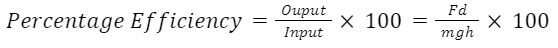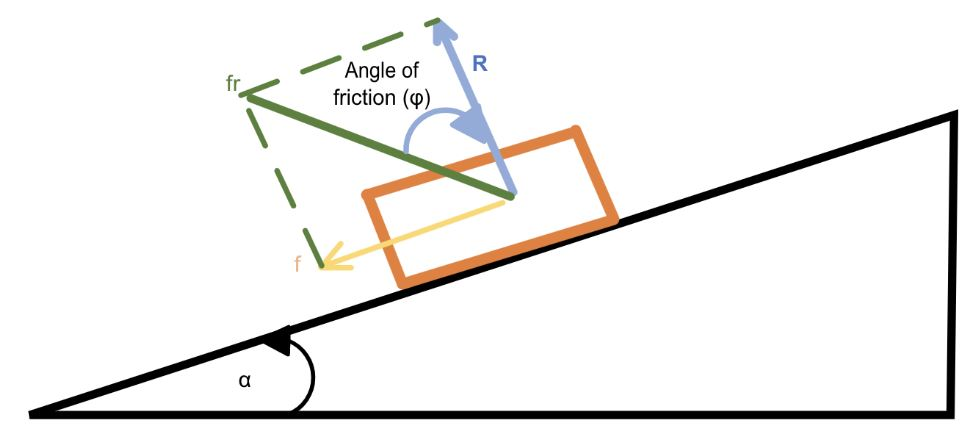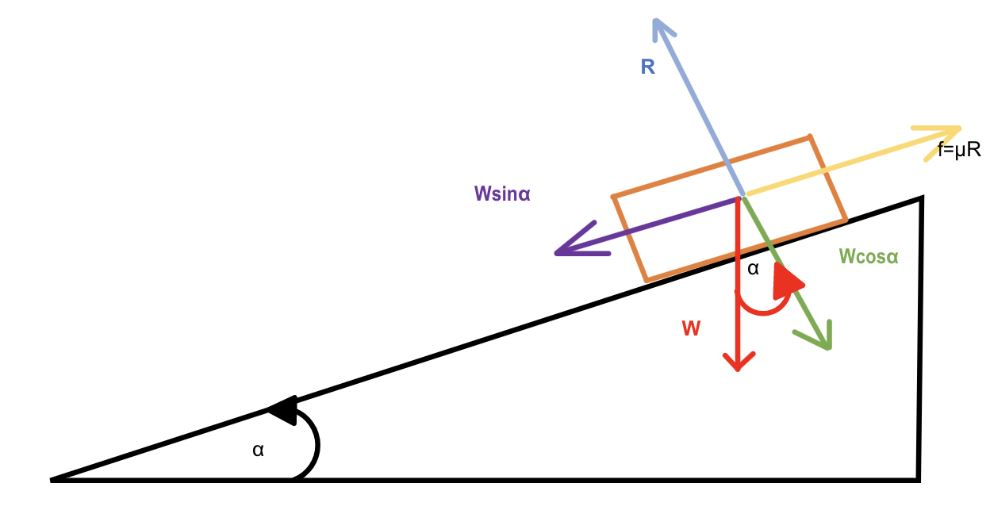Calculation of the efficiency of an inclined plane might look easy to many. Yet, it might be challenging at times.

Although a ramp used at airports appears to be a convenient way to transfer items rather than lift them, understanding the efficiency of an inclined plane can be difficult. At least, it did for me when I was trying to figure out what factors might affect the efficiency of an inclined plane. When employing an inclined plane, lifting items seems to be intuitive. This ease is due to the efficiency of an inclined plane. What is an inclined plane's efficiency, and how can we calculate it?

Efficiency may be explained using two different concepts. First, efficiency is the ratio of output to input. However, the second notion defines an inclined plane's efficiency as the percentage ratio of effort applied in the absence of friction to the effort applied in the presence of friction.

The efficiency of an inclined plane is dependent on many factors, including multiple forces acting on the body resting on an inclined plane. We are going to discuss all these factors later in this topic. First, we will consider an ideal case in which the effects of frictional forces are neglected completely. At the end of the topic, we will explore a realistic approach that requires many more terms and calculations. These measurements will involve frictional force and many other factors. It's going to be very interesting to see that, in some cases, the object on an inclined plane moves upward, sometimes downward, depending on the magnitude and directions of the forces that are acting on them. So let's just keep exploring each concept.

From the perspective and knowledge of a physicist, this article delves into the efficiency of inclined plane measurements.

HideShow

## ‍Let’s cover the basics. Do we need output and input measurements to calculate efficiency?

It would be significantly easier to explain and calculate efficiency mathematically, so let's start with the first concept of measurement efficiency.

We in physics use another term called percentage efficiency, which can be written as follows

Another critical concern is how to compute the output and input. In physics, input is the work done on the machine; in this example, the inclined plane; and output is the work done by the machine. Furthermore, the scientific approach to defining work differs significantly from everyday use; for example, work is said to be done when a specific force applied to an item or simple machine causes it to move a specific distance or spin through an angle.

We can start by understanding output and input. Input is the work done on an object by applying a force F, and this force causes it to cover a distance d, so the work done will be as follows

Here, since work is a scalar or dot product of force F and distance d, that is why cosө is introduced. Also, if force and distance are in the same direction as in our case, then  ө=0. Inserting it into the previous equation, we get,

Now moving to output measurements, here the weight lifted is the force required to lift at a height h.

Inserting values from Equations (4) and ( 5) into Equation (2),we getInserting values from Equations (4) and ( 5) into Equation (2)

## How can we find efficiency in terms of effort?

Before going directly into the second concept of the efficiency of an inclined plane, we need to go through a few terms. First of all, we need to understand two types of angles:

• Angle of friction
• Angle of repose

Angle of friction():

The angle between the reaction force ( R) and the resultant force is between the force of friction and the reaction force (fr). As we can see in the following figure, if there is a body moving upward, then the force of friction will be in the direction opposite to the direction of motion, represented by the yellow color in the following figure, and the normal reaction force ( R) is represented by the blue color. The resultant of f and R is represented by fr, and the angle between fr and R is called the angle of friction.A schematic representation of inclined plane to explain the concept of the angle of friction φ

Figure:2 A schematic representation of inclined plane to explain the concept of the angle of friction φ

Mathematically, we can write as

Angle of repose():

The angle of inclination of a ramp when a resting body is about to slide down it is termed the angle of repose.

If we keep on increasing the angle of inclination of an inclined plane with an object resting on it, then there will come a limit at which, if we increase the angle a bit further, that object will start moving down on an inclined plane. This angle of inclination is called the angle of repose. This limiting angle is called the angle of repose. The body is about to move downwards, so the force of friction would be in the direction shown by the yellow arrow in the following figure.A schematic representation of inclined plane to explain the concept of the angle of repose α

Figure 3: A schematic representation of inclined plane to explain the concept of the angle of repose α

As the body is at rest, all the sum of the horizontal and vertical  forces will be zero in order to satisfy conditions of equilibrium. In other words, the forces applied at right will be equal to the forces applied at left and we get the following equation.

Also, the upward force is equal to the downward force, and it can be written mathematically as follows

Dividing the Equation(9) by the Equation (8), we get

Also, we know that

So we get  μ=tanα

As the tangent of the angle of friction and the tangent of the angle of repose are both equal to the coefficient of friction, We can conclude that both angles are similar to one another. We can write mathematically as follows:

tanφ= μ=tanα

So,  φ=α

How does friction affect the efficiency of an inclined plane?

Friction lowers the efficiency of an inclined plane, but in order to grasp how?

Consider the force F applied at an angle of inclination ( ө),  this force can cause a body to move in the following directions:

• Upward direction
• Downward direction

The direction and magnitude of force will decide the direction of motion. First, we will consider that force is applied in the upward direction that causes the object to move in the upward direction. Now, as clear from the figure, some forces are in the horizontal direction and some are in the vertical direction. Suppose the object is at rest. Then the sum of all the forces would be zero in order to satisfy the first condition of equilibrium. All the forces in the right direction would be equal to the forces in the left direction.

Figure 4: An illustration of an inclined plane with an object resting on it and an illustration of the direction of all possible forces acting on that object.

Similarly, the sum of upward forces will be equal to the sum of downward forces:

We can solve this equation to find the value of R, which is the normal rection force to the weight of the body.

R=Wcosα-Fsinө

We can substitute the value of R from equation (13) into equation (12).

Multiplying every term by cosφ we get

Moving all the terms with F to the right side of the equation, we get:

Taking F as common from the right side of the equation and W from the left side of the equation, we get:

Now if we have the other case in which the instead of going upward object is moving in downward direction the results will remains same except the signs that will change as follows:

Efficiency of an inclined plane:

The efficiency of an inclined plane is the ratio of effort required or needed to move a body on an inclined plane up or down without friction to the effort needed with friction. Mathematically, we can write it as follows:

Figure 5: Representation of an inclined plane with all the terms needed to calculate its efficiency.

Now, as we have discussed earlier, the effort needed in the presence of friction  will be as follows:

Now coming to the effort needed if by some how friction becomes zero or absent than effort will be symbolized as  and is as follows:

Inserting expressions of  and  in equation (16).

Expanding the cos and sin terms by product leads to sum formulas that can be generally written as follows.

cos(a-b)=cosa cosb+sina sinb

Also

sin(a+b)=sina cosb+sinb cosa

So, on expanding the equation (19), we get

Dividing each term in the numerator and denominator by  and we get

Now, since we already know that

So, by inserting it into Equation (20), we get

Equation (21) is the mathematical expression we often use to calculate the efficiency of an inclined plane.